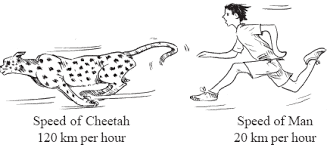1. /
2. CBSE
3. /
4. Class 08
5. /
6. Comparing Quantities worksheet for...

# Comparing Quantities worksheet for class 8### myCBSEguide App

Download the app to get CBSE Sample Papers 2023-24, NCERT Solutions (Revised), Most Important Questions, Previous Year Question Bank, Mock Tests, and Detailed Notes.

## Comparing Quantities worksheet for class 8 Important Topics

• Recalling Ratios and Percentages
• Finding the Increase or Decrease Per cent
• Finding Discounts
• Prices Related to Buying and Selling (Profit and Loss)
• Compound Interest
• Deducing a Formula for Compound Interest
• Rate Compounded Annually or Half Yearly (Semi Annually)
• Applications of Compound Interest Formula## Some important Facts about Comparing Quantities worksheet for class 8

1. Discount is a reduction given on marked price. Discount = Marked Price – Sale Price.
2. Discount can be calculated when discount percentage is given. Discount = Discount % of Marked Price
4. Sales tax is charged on the sale of an item by the government and is added to the Bill Amount. Sales tax = Tax% of Bill Amount
5. Compound interest is the interest calculated on the previous year’s amount (A = P + I)

## NCERT Class 8 Mathematics Solved Worksheets

• Chapter 1: Rational Numbers
• Chapter 2: Linear Equations in One Variable
• Chapter 4: Practical Geometry
• Chapter 5: Data Handling
• Chapter 6: Squares and Square Roots
• Chapter 7: Cubes and Cube Roots
• Chapter 8: Comparing Quantities
• Chapter 9: Algebraic Expressions and Identities
• Chapter 10: Visualising Solid Shapes
• Chapter 11: Mensuration
• Chapter 12: Exponents and Powers
• Chapter 13: Direct and Inverse Proportions
• Chapter 14: Factorisation
• Chapter 15: Introduction to Graphs
• Chapter 16: Playing with Numbers

## Applications of Compound Interest Formula

There are some situations where we could use the formula for calculation of amount in CI.

Here are a few.

1. Increase (or decrease) in population.
2. he growth of a bacteria if the rate of growth is known.
3. The value of an item, if its price increases or decreases in the intermediate years.

To download Printable worksheets for class 8 Mathematics and Science; do check myCBSEguide app or website. myCBSEguide provides sample papers with solution, test papers for chapter-wise practice, NCERT solutions, NCERT Exemplar solutions, quick revision notes for ready reference, CBSE guess papers and CBSE important question papers. Sample Paper all are made available through the best app for CBSE students and myCBSEguide website.### Test Generator

Create question paper PDF and online tests with your own name & logo in minutes.### myCBSEguide

Question Bank, Mock Tests, Exam Papers, NCERT Solutions, Sample Papers, Notes